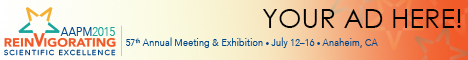# Program Information

## How to Appropriately Calculate Effective Dose for CT Using Either SSDE Or DLP## Presentations

SU-E-I-69 Sunday 3:00PM - 6:00PM Room: Exhibit Hall

Purpose: To demonstrate how to calculate effective dose (E) using specific dose estimates (SSDE), and to correct the current method using CT dose length product (DLP).

Methods: The institutional review board waived the need to obtain informed consent for this analysis. Data were analyzed from 352 chest and 241 abdominopelvic CT images (mean age 6.2 years ± 5.1; range 5-55 kg). To calculate E using SSDE, SSDE was first calculated for chest and abdominopelvic regions. 23 individual organ dose values were estimated using the patient’s weight and effective diameter to look up a conversion factor that was multiplied by patient SSDE. Organ doses were averaged within patient weight-based populations, and used to calculate E by the method of weighted summation of organ dose with ICRP 103 tissue weighting factors (E_ICRP). E was calculated using population averaged CT examination DLP for the chest and abdominopelvic region using k-coefficients published in AAPM Report 96 (E_DLP).

Results: E_DLP was different by an average of 21% (1.4/1.1) in the chest and 42% (2.4/3.4) in the abdominopelvic region as compared to calculating E_ICRP. K-coefficients published in AAPM Report 96 did not account for pitch factor other than unity, were derived using a 32 cm diameter CTDI phantom only, and used ICRP 60 tissue weighting factors. Once corrected for pitch factor, the appropriate size of CTDI phantom, and ICRP 103 tissue weighting factors, E_DLP improved in agreement with E_ICRP to better than 7% (1.4/1.3) and 4% (2.4/2.5) for the chest and abdominopelvic regions, respectively.

Conclusion: Current use of DLP to calculate E was shown to be deficient when compared to the method using ICRP tissue weighting factors due to the outdated means by which the k-coefficients were derived. This study demonstrates a means to calculate E_ICRP using patient SSDE and how to appropriately correct E_DLP.

Contact Email: